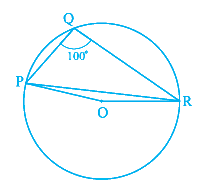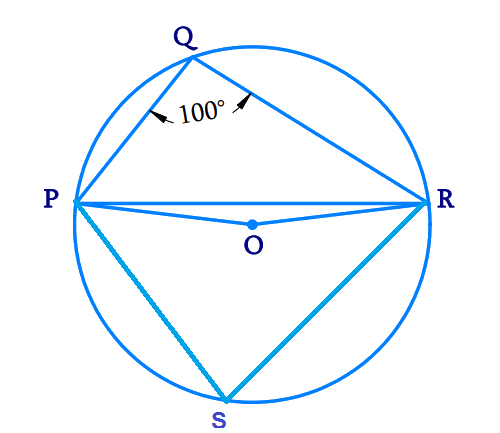In the verge of coronavirus pandemic, we are providing FREE access to our entire Online Curriculum to ensure Learning Doesn't STOP!

# Ex.10.5 Q3 Circles Solution - NCERT Maths Class 9

Go back to  'Ex.10.5'

## Question

In the given figure, \begin {align} \angle {PQR}=100^{\circ} \end {align} where $$P, Q$$ and $$R$$  are points on a circle with center $$O$$ . Find \begin {align}\angle {OPR} \end {align}.Video Solution
Circles
Ex 10.5 | Question 3

## Text Solution

What is known?

$$3$$ points on the circle and

\begin {align}\angle {PQR}=100^{\circ} \end {align}

What is unknown?

Value of $$\angle {PQR}$$

Reasoning:

• The angle subtended by an arc at the centre is double the angle subtended by it at any point on the remaining part of the circle.
• A quadrilateral \begin {align} {ABCD} \end {align} is called cyclic if all the four vertices of it lie on a circle.
• The sum of either pair of opposite angles of a cyclic quadrilateral is \begin {align} 180^{\circ}. \end {align}

Steps:

Mark any point on the major arc side (opposite side to point \begin {align} {Q}\end {align}) as \begin {align} {S.}\end {align}Since all points \begin {align} {P, Q, R, S}\end {align} lie on the circle, \begin {align} {PQRS}\end {align} becomes a cyclic quadrilateral.

We know that, the sum of either pair of opposite angles of a cyclic quadrilateral is \begin {align} 180^\circ.\end {align}

Therefore,

\begin{align} \angle {PQR}+\angle {PSR} &=180^{\circ} \\ 100^{\circ}+\angle {PSR} &=180^{\circ} \\ \angle {PSR} &=180^{\circ}-100^{\circ} \\ &=80^{\circ} \end{align}

We know that, the angle subtended by an arc at the centre is double the angle subtended by it at any point on the remaining part of the circle.

Therefore,

\begin{aligned} \angle {POR} &=2 \angle {PSR} \\ &=2 \times 80^{\circ} \\ &=160^{\circ} \end{aligned}

Consider the \begin{aligned} \Delta {OPR.}\end{aligned} It is an isosceles triangle as

\begin{align} OP &= OR \\ &= \text{Radius of the circle.}\end{align}

\begin {align}∴ \angle {OPR}=\angle {ORP} \end {align}

Sum of all angles in a triangle is \begin {align}180^{\circ}. \end {align}

Therefore,

\begin{align}\angle {OPR}+ \!\angle {POR}+ \! \angle {ORP}&=180^{\circ} \\ \angle {OPR}+160^{\circ}+\angle {OPR}&=180^{\circ} \end{align}

\begin{align} 2 \angle {OPR}&= 180^{\circ} -160^{\circ} \\ \angle {OPR}&=10^{0}\end{align}

Video Solution
Circles
Ex 10.5 | Question 3

Learn from the best math teachers and top your exams

• Live one on one classroom and doubt clearing
• Practice worksheets in and after class for conceptual clarity
• Personalized curriculum to keep up with school